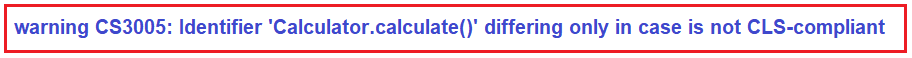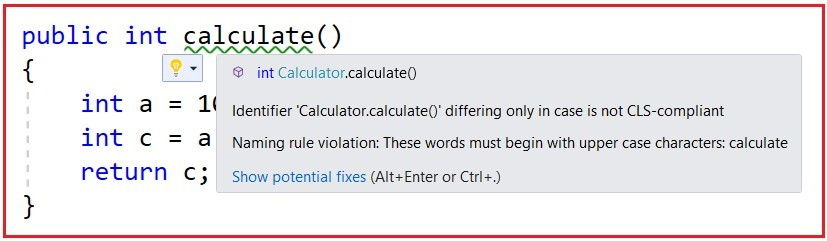# Common Language Specification in .NET Framework

## Common Language Specification (CLS) in .NET Framework

In this article, I am going to discuss Common Language Specification (CLS) in .NET Framework. The Common Language Specification is also called CLS in .NET Framework. Please read our previous article, where we discussed the Common Type System (CTS) in .NET Framework. At the end of this article, you will understand what is Common Language Specification (CLS) and how CLS works in .NET Framework.

##### What is Common Language Specification (CLS) in .NET Framework?

CLS (Common Language Specification) is a part of CLR in the .NET Framework. The .NET Framework supports many programming languages such as C#, VB.NET, J#, F#, etc. Every programming language has its own syntactical rules for writing the code which is known as a language specification. One programming language’s syntactical rules (language specification) cannot be understood by other programming languages. But, there can be situations where we need to communicate between two different programming languages. In order to ensure smooth communication between different .NET Supported Programming Languages, the most important thing is that they should have Common Language Specifications which ensures that language specifications defined in two different languages get compiled into a Common Language Specification.

CLR in .NET Framework will execute all programming language’s code. This is possible because CLR has its own language specification (syntactical rules) which are common to all .NET Supported Programming Languages. At the time of compilation, every language compiler should follow this language specification of CLR and generate the MSIL code. This language specification of CLR is common for all programming languages and this is known as Common Language Specifications (CLS).

In order to understand this concept, what we will do here is, we will violate the common language specifications and then we will see what happens.

##### Example to understand CLS in .NET Framework:

As we know C# is a case-sensitive language whereas VB is not a case-sensitive language. That means in C#, you can declare the same member name multiple times with case differences but it is not possible in VB. So, let us create two class library projects. One uses C# language and the other one uses the VB Programming Language. Then we will try to consume the C# class library project in the VB class library project.

##### Creating C# Class Library Project:

Create a class library project with the name CsharpClassLibrary using the C# programming language. Once you create the C# class library project then add a class file with the name Calculator.cs and then copy and paste the following code into it. As you can see we have created two methods with the same name but with case differences. One method starts with the capital C while the other one starts with a small c.

```namespace CsharpClassLibrary
{
public class Calculator
{
public int Calculate()
{
int a = 10, b = 20;
int c = a + b;
return c;
}

public int calculate()
{
int a = 10, b = 20;
int c = a + b;
return c;
}
}
}
```
##### Creating VB Class Library Project:

To the same CsharpClassLibrary solution, let us add another class library project with the name as VBClassLibrary but using VB as the programming language. Here, in this project we want to use the C# class library project, so first add a reference to the CsharpClassLibrary project. Then create a class with the name TestClass.cs and copy-paste the following code into it.

```Imports CsharpClassLibrary

Public Class TestClass
Public Sub TestMethod()
Dim obj As New Calculator()
obj.Calculate()
End Sub
End Class
```

Now, when you try to build the VB Class Library project, you will get the below error. This is because VB is not case-sensitive and it found two methods with the same name. That means we are violating the Common Language Specifications in the C# code.

‘Calculate’ is ambiguous because multiple kinds of members with this name exist in the class ‘Calculator’.

Now, let us change the second method name to Calculate2 as shown below in the Calculator.cs class file of the CsharpClassLibrary Project.

```namespace CsharpClassLibrary
{
public class Calculator
{
public int Calculate()
{
int a = 10, b = 20;
int c = a + b;
return c;
}

public int Calculate2()
{
int a = 10, b = 20;
int c = a + b;
return c;
}
}
}
```

With the above changes in place, now, build the VB class library project and the build should succeed as expected. Now, you may have one question, how to check whether the code is CLS Compliant or not in .NET Framework?

##### How to check whether the code is CLS Compliant or not in .NET Framework?

In order to check whether your code is following the Common Language Specifications or not in .NET Framework, first, you have to enable CLS Compliant in AssemblyInfo.cs file. So, go to the C# Class Library Project i.e. CsharpClassLibrary Project, and open the AssemblyInfo.cs file which is present inside the Properties folder. Once you open the AssemblyInfo.cs class file, then follow the below 2 steps.

Step1: Import the System namespace as
using System;

Step2: Add the following CLSCompliant attribute at the bottom of this file and set its value to true
[assembly: CLSCompliant(true)]

With the above changes in place in the AssemblyInfo.cs file, now modify the Calculator.cs class file of the CsharpClassLibrary Project as shown below.

```namespace CsharpClassLibrary
{
public class Calculator
{
public int Calculate()
{
int a = 10, b = 20;
int c = a + b;
return c;
}

public int calculate()
{
int a = 10, b = 20;
int c = a + b;
return c;
}
}
}
```

Now, when you build the C# Class Library Project, you will get the following warning.If you modify the AssemblyInfo.cs class file to enable CLSCompliant, then it will be applicable to all the classes within our project. But if you want to enable to CLSCompliant in specific classes, then you can use the CLSCompliant attribute. For example, you can decorate the Calculator class as shown below to enable CLSCompliant only for the Calculator class.

```using System;
[assembly: CLSCompliant(true)]
namespace CsharpClassLibrary
{
public class Calculator
{
public int Calculate()
{
int a = 10, b = 20;
int c = a + b;
return c;
}

public int calculate()
{
int a = 10, b = 20;
int c = a + b;
return c;
}
}
}```

Now, you can see, the calculate method (starting with small c) gives one warning and says it is not CLS-compliant as shown in the below image.Note: CLS (Common Language Specification) in .NET Framework defines a set of rules and restrictions that every language must follow which runs under the .NET framework. The languages which follow the CLS Specifications are said to be CLS Compliant. In simple words, we can say that CLS in .NET Framework enables Cross-Language Integration or Interoperability.

In the next article, I am going to discuss the Managed and Unmanaged Code in .NET Applications in detail. Here, in this article, I try to explain Common Language Specification (CLS) in C#.NET Framework in detail and I hope you enjoy CLS in the C# article.

### 1 thought on “Common Language Specification in .NET Framework”

1.This information is very useful for me understand this topic .
Thank u so much